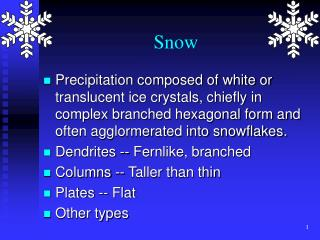DownloadDownload PresentationSnow

# Snow

Télécharger la présentation## Snow

- - - - - - - - - - - - - - - - - - - - - - - - - - - E N D - - - - - - - - - - - - - - - - - - - - - - - - - - -
##### Presentation Transcript

1. Snow • Precipitation composed of white or translucent ice crystals, chiefly in complex branched hexagonal form and often agglormerated into snowflakes. • Dendrites -- Fernlike, branched • Columns -- Taller than thin • Plates -- Flat • Other types

2. Snow • Snow shapes, or habits, are temperature dependent. Temperature (C)Crystal Habit 0 to -4 Thin Plates -4 to -10 Columns -10 to -12 Plates -12 to -16 Dendrites -16 to -22 Plates Much of the snow we see are aggregates of these crystal habits.

3. Snow • The warmer the air, but still below freezing, often the heavier the snowfall. • Warmer temperatures have a higher saturation vapor pressure so there are more vapor molecules that can allow crystals to grow. • Greater chance of large aggregates of crystals forming in warmer temperatures.

4. Snow • Snow Shower: A form of precipitation from a convective cloud that is short in duration, starts and stops suddenly, and often has a rapid change in intensity. • Flurries: A very light and usually brief show shower. • Snow Squall: A sudden wind associated with snowfall that occurs suddenly and is usually intense and short lived.

5. Freezing Rain • Precipitation that falls to the ground as a liquid then freezes upon impact with the ground or exposed objects. Forms a glaze of ice on the objects and ground.

6. Freezing Rain Cold: T < 0C Warm: T > 0C Rain freezes upon contact with below freezing surface. Shallow freezing layer

7. Sleet -- Ice Pellets Cold: T < 0C Warm: T > 0C Rain freezes before reaching the surface. Deep freezing layer

8. Measuring Precipitation • Rain Gauge • Standard • Weighing Gauge • Tipping Bucket • Radar • Optical Rain Gauge

9. State Variables • State variables are quantities that tell us the state of a gas -- the atmosphere. Several of these deserve special attention: • Temperature • Density • Pressure

10. State Variables -- Temperature • Temperature: The temperature of a substance is a measure of the average kinetic energy of the molecules in that substance. Thus atmospheric temperature is proportional to the speed of the air molecules.

11. Temperature T ~ mV2, or as T , V Records: Low: -1270 F in Vostok ,Antarctica, High: + 1360 F in El Azizia, Libya.

12. Temperature T1 > T2 m1v12 > m2v22 (Kinetic Energy) If m1 = m2 then: v1 > v2

13. Temperature Scales • Three (3) temperature scales and their units: • Fahrenheit (F) -- German • Celsius (C) -- Swedish • Absolute (K) -- “Scientific”

14. Density • The density of a substance is defined as the amount of mass of a substance in a given volume. • It can also be defined by a number density that tells us the number of “things” in a given volume. • Number of students in M204 • Number of water drops in a cubic centimeter of cloud

15. Density Unit mass V1 = V2 m1 < m2 m1/v1 < m2 /v2 1 < 2

16. Pressure • The air pressure is the force per unit area that the atmosphere exerts on any surface it touches. • The molecules of the air are in constant rapid motion. • When a molecule collides with a surface, such as your skin, the molecule exerts a force on that surface.

17. Pressure • Pressure is the cumulative effect of these air molecules colliding with a surface. Air Pressure Depends On: The mass of the molecules Gravity The kinetic molecular activity (temperature)

18. Pressure • Pressure is the force per unit area: • In meteorology we us the units millibars • “Typical” sea-level pressure is approximately 1013 mb. F P = A

19. Ideal Gas Law • We have now investigated three state variables: • Temperature • Pressure • Density • These variables change from place to place and in time but they do not do so independently. • This interrelationship is known as the Ideal Gas Law.

20. Ideal Gas Law: P = R  T • If the density is constant • If T increases then p increases • If T decreases then p decreases • If the temperature is constant • If p increases then  increases • If p decreases then  decreases • If the pressure is constant • If T increases then  decreases • If T decreases then  increases

21. Ideal Gas Law: Reprise • We can substitute the definition for density to put the ideal gas law into terms that we can easily measure: temperature, pressure, volume. m  = P = R  T V PV = m R T

22. Ideal Gas Law: Reprise • We see that for a given mass of gas: • For a constant p, T increases, V increases • For a constant V, T increases, p increases • For a constant T, p increases, V decreases PV = constant T

23. Pressure Balanced by air pressure. Gravity But, the air doesn’t fall down!!! Pressure: Force due to gravity that the mass of the atmosphere exerts on a unit area of surface.